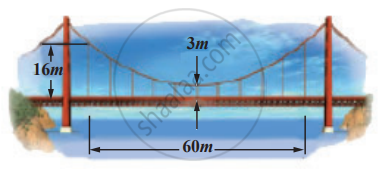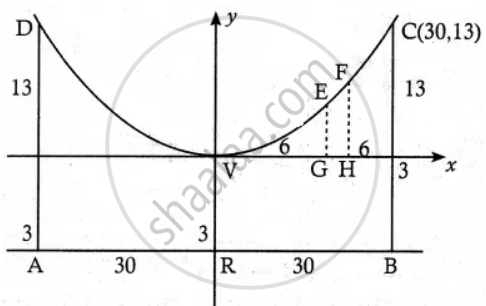Tamil Nadu Board of Secondary EducationHSC Arts Class 12th

# Parabolic cable of a 60 m portion of the roadbed of a suspension bridge are positioned as shown below. Vertical Cables are to be spaced every 6m along this portion of the roadbed. Calculate - Mathematics

Diagram
Sum

Parabolic cable of a 60 m portion of the roadbed of a suspension bridge are positioned as shown below. Vertical Cables are to be spaced every 6m along this portion of the roadbed. Calculate the lengths of first two of these vertical cables from the vertex.#### SolutionFrom the diagram,

The equation is x2 = 4 ay and it passes through C(30, 13)

Equation of Parabola x2 = 4ay.

302 = 4a × 13

4a = 30^2/13

∴ Equation of the parabola is

x2 = 30^2/13 y

(i) Let VG = 6 and GE = y

∴ E is (6, y) and it lies on the parabola

36 = 30^2/13 y

⇒ y = 0.52

Gable from the road = 3 + 0.52

= 3.52 m

(ii) Let VH = 12 and HF = y

∴ F(12, y) lies on the parabola

122 = 30^2/13  y

⇒ y = (144 xx 13)/900

= 208/100 y

y = 2.08

Cable from the road = 3 + 2.08 = 5.08

The heights of the first two vertical cables from the vertex are 3.52 m and 5.08 m

Concept: Real Life Applications of Conics
Is there an error in this question or solution?

#### APPEARS IN

Tamil Nadu Board Samacheer Kalvi Class 12th Mathematics Volume 1 and 2 Answers Guide
Chapter 5 Two Dimensional Analytical Geometry-II
Exercise 5.5 | Q 5 | Page 214
Share